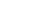加入国际站

铜币： 剩余 * 评分才能提交噢 你这么厉害圈妹造吗！ 亲，给你个好评哦！ 地球已经无法阻止你的脚步了! 我要代表圈妹奖励你么么哒一个！ 在外贸圈上又发现一个牛人！希望继续分享！ 你造福各位外贸网友啦！ 你的帖子太TM牛了！ 谢谢转载，送人玫瑰手留余香
|

• 0 楼#

假相：顾客是上帝；所有的顾客都一样。
真相：顾客不是上帝；对顾客要区别对待。

顾客和客户是一回事吗？尽管我们经常不加区分地使用这两个词，严格说起来，这两个词是不同的，其中的区别还有深意。

著名的麦肯锡咨询公司的创始人鲍尔说：“我们没有顾客（customer），我们只有客户（client）。”他的下一句话点出了原因：“我们不属于哪个行业（industry），我们自成一个专业（profession）。”

一般说来，专业服务——包括律师、会计师、建筑师、广告代理、管理咨询等——的使用者，被称作客户；而普通的商品和服务的使用者，被称为顾客。
显然，专业服务提供者跟客户之间的关系，比普通的厂商跟顾客之间的关系，要更深厚、更紧密。权威的Merriam-Webster词典对“客户”的第一个释义是“在另一个人保护之
下的人”，对“顾客”的第一个释义是“购买一种商品或服务的人”。

比较这两个定义，更能看出：客户和顾客的本质不同，不在于使用的是否是专业服务，而在于——你跟客户的关系，是你照料和保护他的利益；而你跟顾客的关系，只是你把东西（不管是产品还是服务）卖给了他。

也就是说，即使你是专业服务的提供商，你也可能只是把服务的对象当作了顾客。即使你不是专业服务的提供商，不是律师行、会计师行什么的，而只是卖电脑的或者开商场
的，但是你做到了照料和保护对方的利益，发展起了长期合作、信任的关系，你就把对方变成了客户。

显然，客户总是比顾客少。一家管理咨询公司，客户可能只有两位数；一家饮料公司，顾客则可能上千万。因此可以这么说，单个客户比单个顾客更重要。但是，这并不意味着客
户比顾客更是上帝，你更应该百依百顺，而是说：选择客户比选择顾客需要更谨慎，在很多时候，你要拒绝他成为你的客户！

一、偏离本行的客户要拒绝。
二、偏离核心价值的客户要拒绝。

三、自己不信任的客户要拒绝。

四、打击自己员工士气的客户要拒绝。

五、无利可图的客户要拒绝。

六、太大的客户要拒绝。

• 1 楼#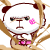• 2 楼#

看看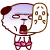• 3 楼#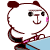• 4 楼#

被LZ骗了，里面什么都没有！FFFFFFFFFFFFFFFFFFFFFFFFFFFFFFFFFFFFFFFFFFFFFFFFFFFFFFFFFFFFFFFFFFFFFFFFFFFFFFFFFFFFFFFFFFFFFFFFFFFFFFFFFFFFFFFFFFFFFFFFFFFFFFFFFFFFFFFFFFFFFFFFFFFFFFFFFFFFFFFFFFFFFFFFFFFFFFFFFFFFFFFFFFFFFFFFFFFFFFFFFFFFFFFFFFFFFFFFFFFFFFFF

• 5 楼#

用户被禁言,该主题自动屏蔽!

• 6 楼#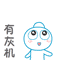• 7 楼#• 8 楼#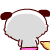• 9 楼#• 10 楼#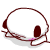• 11 楼#

cn1000479915:被LZ骗了，里面什么都没有！FFFFFFFFFFFFFFFFFFFFFFFFFFFFFFFFFFFFFFFFFFFFFFFFFFFFFFFFFFFFFFFFFFFFFFFFFFFFFFFFFFFFFFFFFFFFFFFFFFFFFFFFFFFFFFFFFFFFFFFFFFFFFFFFFFFFFFFFFFFFFFFFFFFFFFFFFFFFFFFFFFFFFFFFFFFFFFFFFFFFFFFFFFFFFFFFFFFFFFFFFFFFFFFFFFFFFFFFFFFFF .. (2012-11-11 13:30)有的，是你自己没看到吧

• 12 楼#• 13 楼#

我来给大家大家鉴定下有没有内容

• 14 楼#看看

• 15 楼#

好东西，顶一个

• 16 楼#

看看呗

• 17 楼#

看一下

• 18 楼#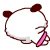• 19 楼#

楼主的菊花晶莹剔透，心中一定还有梦~

•
假相：顾客是上帝；所有的顾客都一样。
真相：顾客不是上帝；对顾客要区别对待。

顾客和客户是一回事吗？尽管我们经常不加区分地使用这两个词，严格说起来，这两个词是不同的，其中的区别还有深意。

著名的麦肯锡咨询公司的创始人鲍尔说：“我们没有顾客（customer），我们只有客户（client）。”他的下一句话点出了原因：“我们不属于哪个行业（industry），我们自成一个专业（profession）。”

一般说来，专业服务——包括律师、会计师、建筑师、广告代理、管理咨询等——的使用者，被称作客户；而普通的商品和服务的使用者，被称为顾客。
显然，专业服务提供者跟客户之间的关系，比普通的厂商跟顾客之间的关系，要更深厚、更紧密。权威的Merriam-Webster词典对“客户”的第一个释义是“在另一个人保护之
下的人”，对“顾客”的第一个释义是“购买一种商品或服务的人”。

比较这两个定义，更能看出：客户和顾客的本质不同，不在于使用的是否是专业服务，而在于——你跟客户的关系，是你照料和保护他的利益；而你跟顾客的关系，只是你把东西（不管是产品还是服务）卖给了他。

也就是说，即使你是专业服务的提供商，你也可能只是把服务的对象当作了顾客。即使你不是专业服务的提供商，不是律师行、会计师行什么的，而只是卖电脑的或者开商场
的，但是你做到了照料和保护对方的利益，发展起了长期合作、信任的关系，你就把对方变成了客户。

显然，客户总是比顾客少。一家管理咨询公司，客户可能只有两位数；一家饮料公司，顾客则可能上千万。因此可以这么说，单个客户比单个顾客更重要。但是，这并不意味着客
户比顾客更是上帝，你更应该百依百顺，而是说：选择客户比选择顾客需要更谨慎，在很多时候，你要拒绝他成为你的客户！

一、偏离本行的客户要拒绝。
二、偏离核心价值的客户要拒绝。

三、自己不信任的客户要拒绝。

四、打击自己员工士气的客户要拒绝。

五、无利可图的客户要拒绝。

六、太大的客户要拒绝。

•• 看看•• 被LZ骗了，里面什么都没有！FFFFFFFFFFFFFFFFFFFFFFFFFFFFFFFFFFFFFFFFFFFFFFFFFFFFFFFFFFFFFFFFFFFFFFFFFFFFFFFFFFFFFFFFFFFFFFFFFFFFFFFFFFFFFFFFFFFFFFFFFFFFFFFFFFFFFFFFFFFFFFFFFFFFFFFFFFFFFFFFFFFFFFFFFFFFFFFFFFFFFFFFFFFFFFFFFFFFFFFFFFFFFFFFFFFFFFFFFFFFFFFF

• 用户被禁言,该主题自动屏蔽!

•••••• cn1000479915:被LZ骗了，里面什么都没有！FFFFFFFFFFFFFFFFFFFFFFFFFFFFFFFFFFFFFFFFFFFFFFFFFFFFFFFFFFFFFFFFFFFFFFFFFFFFFFFFFFFFFFFFFFFFFFFFFFFFFFFFFFFFFFFFFFFFFFFFFFFFFFFFFFFFFFFFFFFFFFFFFFFFFFFFFFFFFFFFFFFFFFFFFFFFFFFFFFFFFFFFFFFFFFFFFFFFFFFFFFFFFFFFFFFFFFFFFFFFF .. (2012-11-11 13:30)有的，是你自己没看到吧

•• 我来给大家大家鉴定下有没有内容

•看看

• 好东西，顶一个

• 看看呗

• 看一下

•• 楼主的菊花晶莹剔透，心中一定还有梦~

375 回复